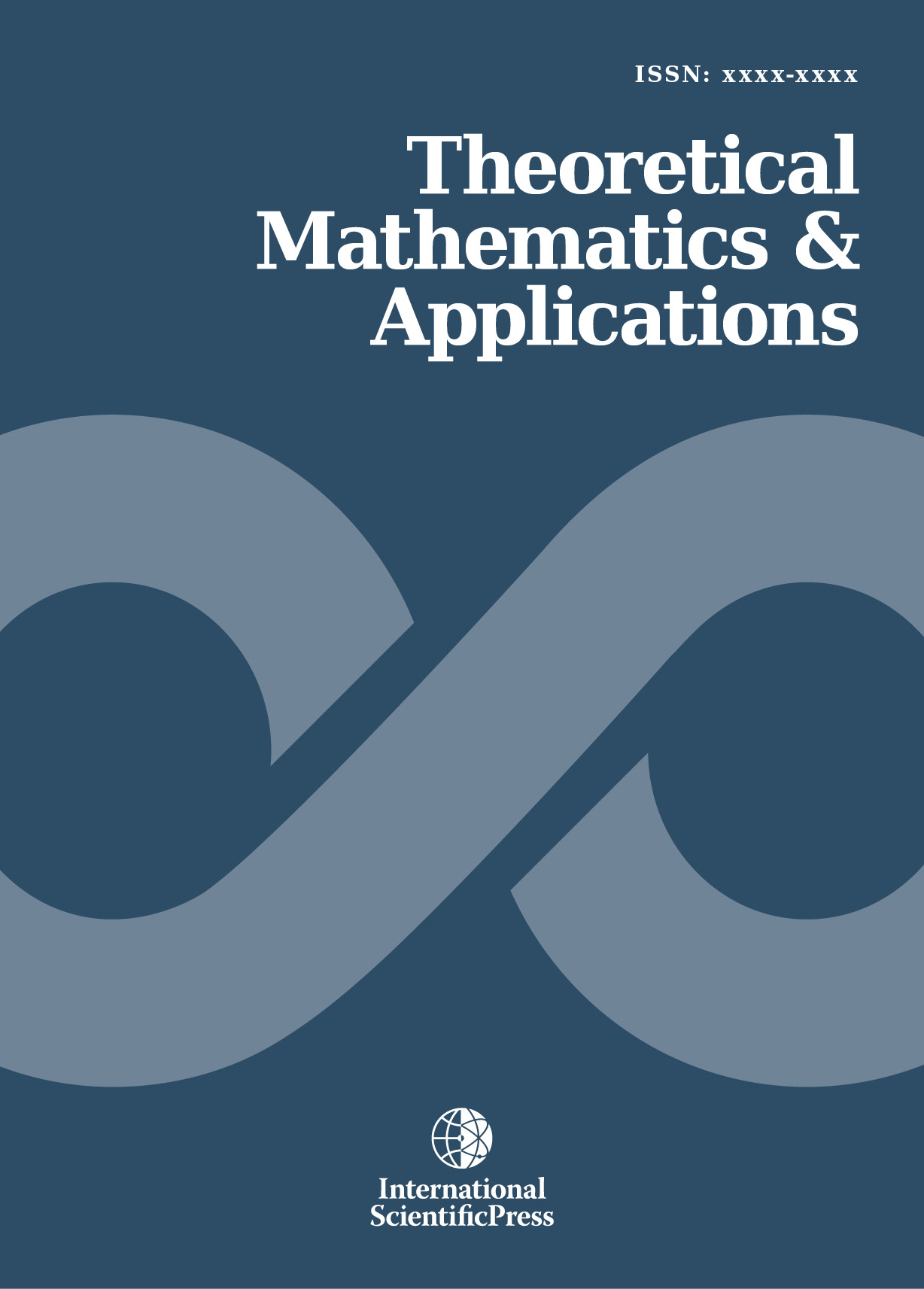# Theoretical Mathematics & Applications

#### Proof of Bunyakovsky's conjecture

•[ Download ]
• Abstract

In 1857, twenty years after Dirichlet's theorem on arithmetic progressions, the conjecture of the Ukrainian mathematician Victor Y. Bunyakovsky (1804-1889) is already a try to generalize this theorem to polynomial integer functions of degree m>1. This conjecture states that under three conditions a polynomial integer function of degree m>1 generates infinitely many primes.

The main contribution of this paper is to introduce a new approach to this conjecture. The key ideas of this new approach is to relate the conjecture to a general theory (here arithmetic progressions) and use the active constraint of this theory (Dirichlet's theorem) to achieve the proof.

Mathematics Subject Classification: 11A41, 11A51, 11B25, 11C08.

Keywords: Bunyakovsky, Polynomials, Dirichlet, Arithmetic Progression, Prime.ISSN: 1792-9687 (Print)
1792-9709 (Online)